# An alpha-particle has a charge of +2e and a mass of 6.64 * 10^-27 kg. It...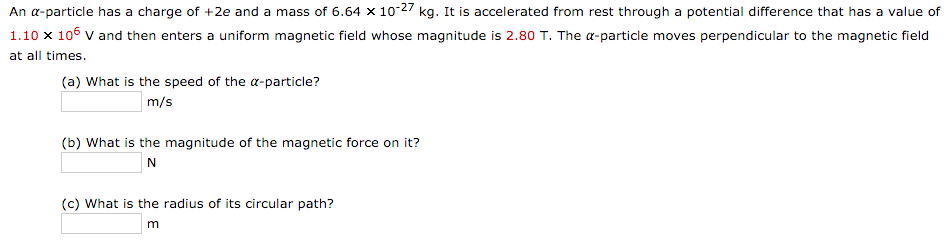An alpha-particle has a charge of +2e and a mass of 6.64 * 10^-27 kg. It is accelerated from rest through a potential difference that has a value of 1.10 * 10^6 V and then enters a uniform magnetic field whose magnitude is 2.80 T. The alpha-particle moves perpendicular to the magnetic field at all times. What is the speed of the alpha-particle What is the magnitude of the magnetic force on it What is the radius of its circular path

Data Given Charge on the alpha particle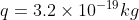, Mass of the alpha particle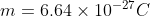Potential Difference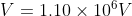And Magnetic field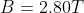(a) For the speed of alpha particle

We know that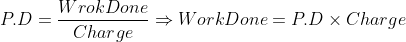From work energy theorem Work done = Change in K.E

So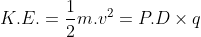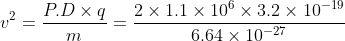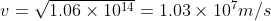(b)

Magnetic Force on the alpha particle is given by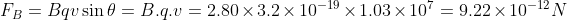(c) Radius of Circular Path is given by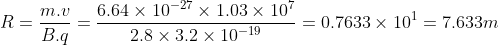#### Earn Coin

Coins can be redeemed for fabulous gifts.

Similar Homework Help Questions
• ### An alpha-particle has a charge of +2e and a mass of 6.64 times 10^-27 kg. It...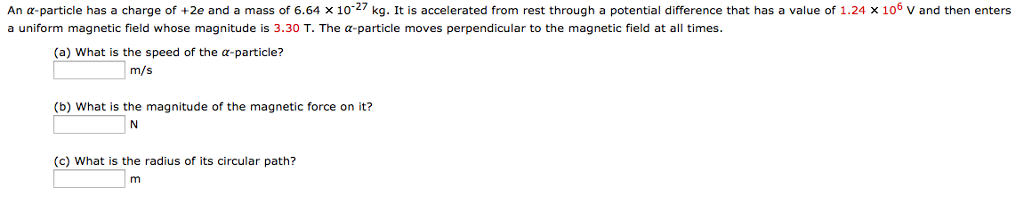An alpha-particle has a charge of +2e and a mass of 6.64 times 10^-27 kg. It is accelerated from rest through a potential difference that has a value of 1.24 times 10^6 v and then enters a uniform magnetic field whose magnitude is 3.30 T. The alpha-particle moves perpendicular to the magnetic field at all times. (a) What is the speed of the alpha-particle? m/s (b) What is the magnitude of the magnetic force on it? N (c) What is...

• ### An α-particle has a charge of +2e and a mass of 6.64 × 10-27 kg. It...

An α-particle has a charge of +2e and a mass of 6.64 × 10-27 kg. It is accelerated from rest through a potential difference that has a value of 1.09 × 106 V and then enters a uniform magnetic field whose magnitude is 2.63 T. The α-particle moves perpendicular to the magnetic field at all times. What is (a) the speed of the α-particle, (b) the magnitude of the magnetic force on it, and (c) the radius of its circular...

• ### An α-particle has a charge of +2e and a mass of 6.64 × 10-27 kg. It...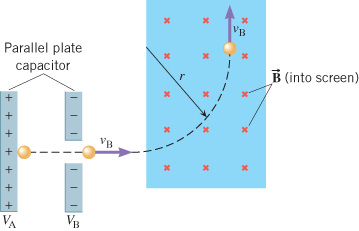An α-particle has a charge of +2e and a mass of 6.64 × 10-27 kg. It is accelerated from rest through a potential difference that has a value of 1.30 × 106 V and then enters a uniform magnetic field whose magnitude is 1.14 T. The α-particle moves perpendicular to the magnetic field at all times. What is (a) the speed of the α-particle, (b) the magnitude of the magnetic force on it, and (c) the radius of its circular...

• ### An α-particle has a charge of +2e and a mass of 6.64 10-27 kg. It is...

An α-particle has a charge of +2e and a mass of 6.64 10-27 kg. It is accelerated from rest through a potential difference that has a value of 1.08 106 V and then enters a uniform magnetic field whose magnitude is 2.30 T. The α-particle moves perpendicular to the magnetic field at all times. (a) What is the speed of the α-particle? m/s (b) What is the magnitude of the magnetic force on it? N (c) What is the radius...

• ### A. An α-particle has a charge of +2e and a mass of 6.64 × 10-27 kg....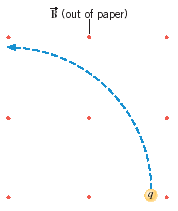A. An α-particle has a charge of +2e and a mass of 6.64 × 10-27 kg. It is accelerated from rest through a potential difference that has a value of 1.67 × 106 V and then enters a uniform magnetic field whose magnitude is 2.49 T. The α-particle moves perpendicular to the magnetic field at all times. What is (a) the speed of the α-particle, (b) the magnitude of the magnetic force on it, and (c) the radius of its...

• ### Chapter 21, Problem 19 An a-particle has a charge of +2e and a mass of 6.64...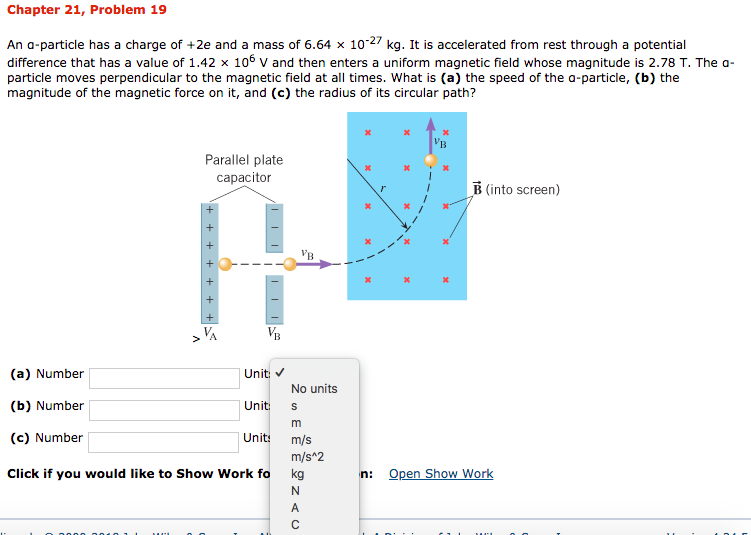Chapter 21, Problem 19 An a-particle has a charge of +2e and a mass of 6.64 x 10-27 kg. It is accelerated from rest through a potential difference that has a value of 1.42 x 10° V and then enters a uniform magnetic field whose magnitude is 2.78 T. The a particle moves perpendicular to the magnetic field at all times. What is (a) the speed of the a-particle, (b) the magnitude of the magnetic force on it, and (c)...

• ### A proton (charge le, mass mp) and an alpha particle (charge 2e, mass 4mp) in a...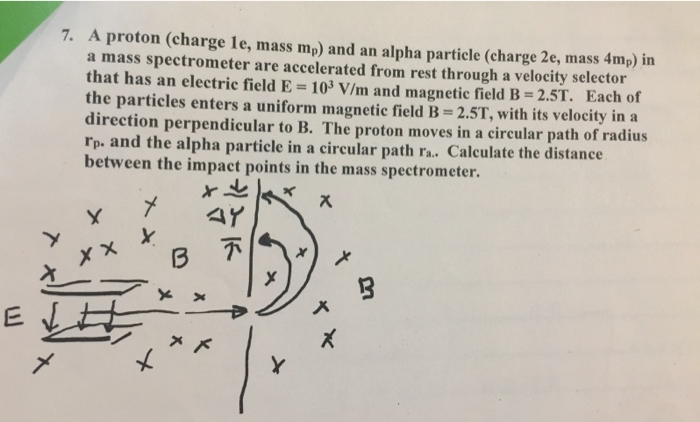A proton (charge le, mass mp) and an alpha particle (charge 2e, mass 4mp) in a mass spectrometer are accelerated from rest through a velocity selector that has an electric field E 103 V/m and magnetic field B- 2.5T. Each of the particles enters a uniform magnetic field B-2.5T, with its velocity in a direction perpendicular to B. The proton moves in a circular path of radius rp. and the alpha particle in a circular path ra. Calculate the distance...

• ### Fall 2018 Physic 122 An a-particle has a charge of +2e and a mass of 6.64...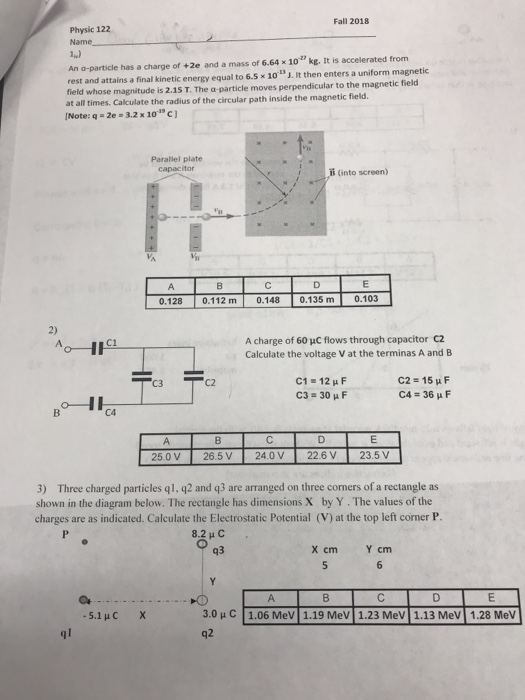Fall 2018 Physic 122 An a-particle has a charge of +2e and a mass of 6.64 x 10 kg. It is accelerated from rest and attains a final kinetic energy equal to 6.5 x 10 1 J. It then enters a uniform magnetic field whose magnitude is 2.15 T. The ?-particle moves perpendicular to the magnetic field at all times. Calculate the radius of the circular path inside the magnetic field. [Note: q-2e-3.2 x 101, C] Parallel plate capacitor ii...

• ### An alpha particle of charge +2e and mass 6.64 × 10-27 kg is moving in the...

An alpha particle of charge +2e and mass 6.64 × 10-27 kg is moving in the +x direction at 1.03×106 m/s. It enters a region where the electric field is 2835 N/C in the -x direction. How far will the alpha particle travel before it comes to a stop? How much time will elapse from when the alpha particle enters the electric field to when it stops?

• ### An alpha particle (mass = 6.64⨯10-27 kg, charge = +2e) is accelerated by a potential drop...

An alpha particle (mass = 6.64⨯10-27 kg, charge = +2e) is accelerated by a potential drop V = -40 kV. What is its velocity upon emerging from the accelerating region?